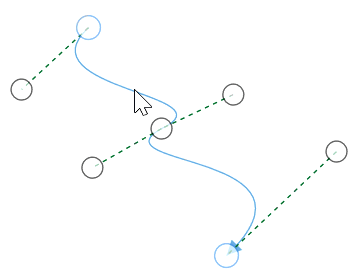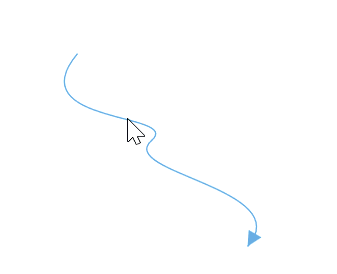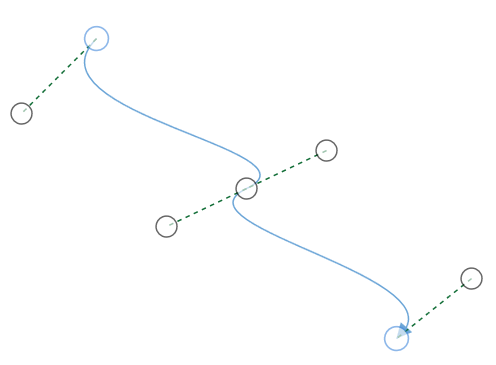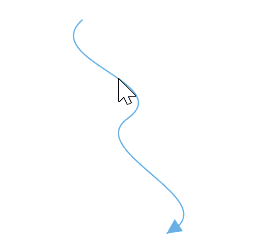# How to interact with cubic bezier segments efficiently

3 Sep 20204 minutes to read

While interacting with multiple cubic bezier segments, you can maintain their control points in same distance and angle by using BezierSmoothness property of `CubicCurveSegment` class.

BezierSmoothness value Description Output
SymmetricDistance Both segments intersecting control points will be in same distance when any one them is editingSymmetricAngle Both segments intersecting control points will be in same angle when any one them is editingSymmetric Both segments intersecting control points will be in same angle and same distance when any one them is editingNone Segemnts control points are independent to each other``````<!--create the connector with cubic curve segments-->
<syncfusion:ConnectorViewModel SourcePoint="100,100" TargetPoint="300,300">
<syncfusion:ConnectorViewModel.Segments>
<syncfusion:ConnectorSegments>
<syncfusion:CubicCurveSegment BezierSmoothness="SymmetricDistance"
Constraints="None" Point1="50,150"
Point2="260,150" Point3="200,200"/>
<syncfusion:CubicCurveSegment BezierSmoothness="SymmetricDistance"
Constraints="None" Point1="100,250"
Point2="350,260" />
</syncfusion:ConnectorSegments>
</syncfusion:ConnectorViewModel.Segments>
</syncfusion:ConnectorViewModel>``````
``````//create the connector with cubic bezier segments
ConnectorViewModel cubicBezierConnector = new ConnectorViewModel()
{
SourcePoint = new Point(100, 100),
TargetPoint = new Point(300, 300),
Segments = new ObservableCollection<IConnectorSegment>()
{
//Specify the segment as cubic curve segment
new CubicCurveSegment()
{
//Specify the bezier smoothness value
BezierSmoothness = BezierSmoothness.SymmetricDistance,
Constraints = SegmentConstraints.None,
Point1 = new Point(50,150),
Point2 = new Point(260,150),
Point3 = new Point(200,200),
},
new CubicCurveSegment()
{
//Specify the bezier smoothness value
BezierSmoothness = BezierSmoothness.SymmetricDistance,
Constraints = SegmentConstraints.None,
Point1 = new Point(100,250),
Point2 = new Point(350,260),
}
}
};``````